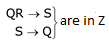# GATE Solved Paper 2017-19 - GATE 2019

• A

{wwR |w ∈ {a,b}*}• B

{wanbnwR |w {a, b}*, n ≥ 0}• C

{wanwRbn |w {a,b}*, n ≥ 0}• D

{anbi |i ∈ {n, 3n, 5n}, n ≥ 0}• Option : C
• Explanation :
(a) {wwR |w ∈ {a, b}* } is a CFL

(b) {wan bn wR |w ∈ {a, b}*, n ≥ 0} is a CFL, since we can first push w, then a’s, b’s pop with a’s and wR pops with the w. So PDA can accept the language.

(c) {wanwR bn|w ∈ {a, b}*, n ≥ 0} is a not CFL because after pushing w, we need to push a’s into stack which will stop the w from being matched with wR. If we don’t push a’s after w, than later we cannot match with bn. So this language is not acceptable by a PDA and hence not a CFL.

(d) {anbi |i ∈ {n, 3n, 5n}, n ≥ 0} = anbn∪ anb3n∪ anb5n is CFL since each of the three parts is a CFL and closure under union guarantees that result also is a CFL.

• A

Both I and II• B

I only• C

II only• D

Neither I nor II• Option : C
• Explanation :
X(PQRS) {QR → S, R → P, S → Q} decomposed into  Y(PR) Z(QRS) {R → P} {QR → S, S → Q} Candidate key : R Candidate key : QR, RS Relation Y in BCNF Relation Z in 3NF but not BCNF
The common attribute between Y and Z relations is R which is key for relation Y.
So that given decomposition is lossless join decomposition.
R → P in Yand dependency preserving decomposition.
Hence, C is the correct answer.

• A

16 × 210• B

256 × 210• C

4 × 220• D

8 × 220• Option : B
• Explanation :
1 word = 4 bytes
Page size = 8 kB = 213 B
Number of words in 1 page = 213/22 = 211
TLB can hold 128 valid entries so, at most 128 × 211 memory address can be addressed without TLB miss.
128 × 211 = 256 × 210

• A

S1 and S2• B

S3 and S4• C

S2 and S3• D

S1 and S4• Option : B
• Explanation :
S1: The set : LRE is known to be countably infinite since it corresponds with set of turing machines.
S2: Since syntactically valid C programs surely run on Turing machines, this set is also : a subset of set of Turing machines, which is countable.
S3: Set of all languages = 2Σ which is known to be uncountable. Σ* countably infinite
⇒ 2Σ is uncountable.
S4: Set of all non-regular languages includes set : L NOT RE which is uncountable infinite and hence is uncountable.
So, S3 and S4 are uncountable.

• A

S1 and S2• B

S1 and S3• C

S2 and S3• D

S1, S2 and S3• Option : C
• Explanation :
∀x[∀z⊗x ⇒ ((z = x) ∨ (z = 1)) ⇒ ∃w (w > x) ∧ (∀z z⊗w ⇒ ((w = z) ∨ (z = 1)))]

The predicate ϕ simply says that if z is a prime number in the set then there exists another prime number is the set which is larger.

Clearly ϕ is true in S2 and S3 since in set of all integers as well as all positive integers, there is a prime number greater than any given prime number.

However, in S1 : {1, 2, 3, .....100} ϕ is false since for prime number 97 ∈ S1 there exists no prime number in the set which is greater. So correct answer is C.
Related Quiz.
GATE 2019